# 3. A student sits on a freely rotating stool holding two dumbbells, each of mass 2.92...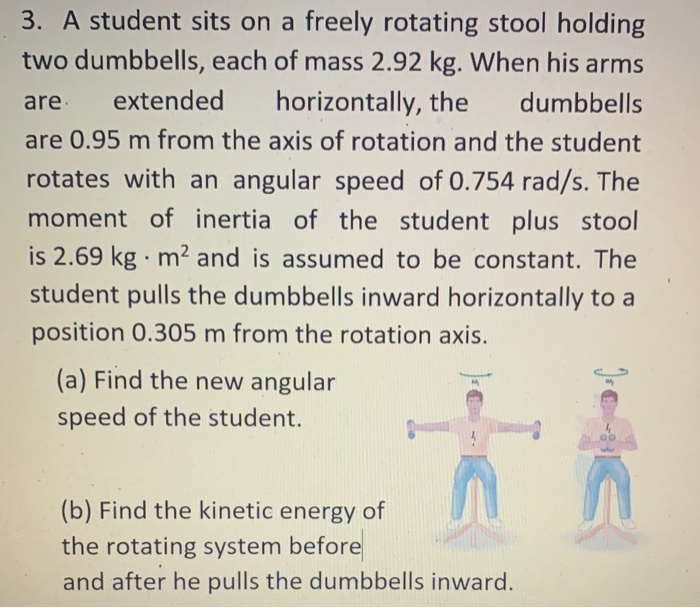3. A student sits on a freely rotating stool holding two dumbbells, each of mass 2.92 kg. When his arms are extended horizontally, the dumbbells are 0.95 m from the axis of rotation and the student rotates with an angular speed of 0.754 rad/s. The moment of inertia of the student plus stool is 2.69 kg · m2 and is assumed to be constant. The student pulls the dumbbells inward horizontally to a position 0.305 m from the rotation axis. (a) Find the new angular speed of the student. Q (b) Find the kinetic energy of the rotating system before and after he pulls the dumbbells inward.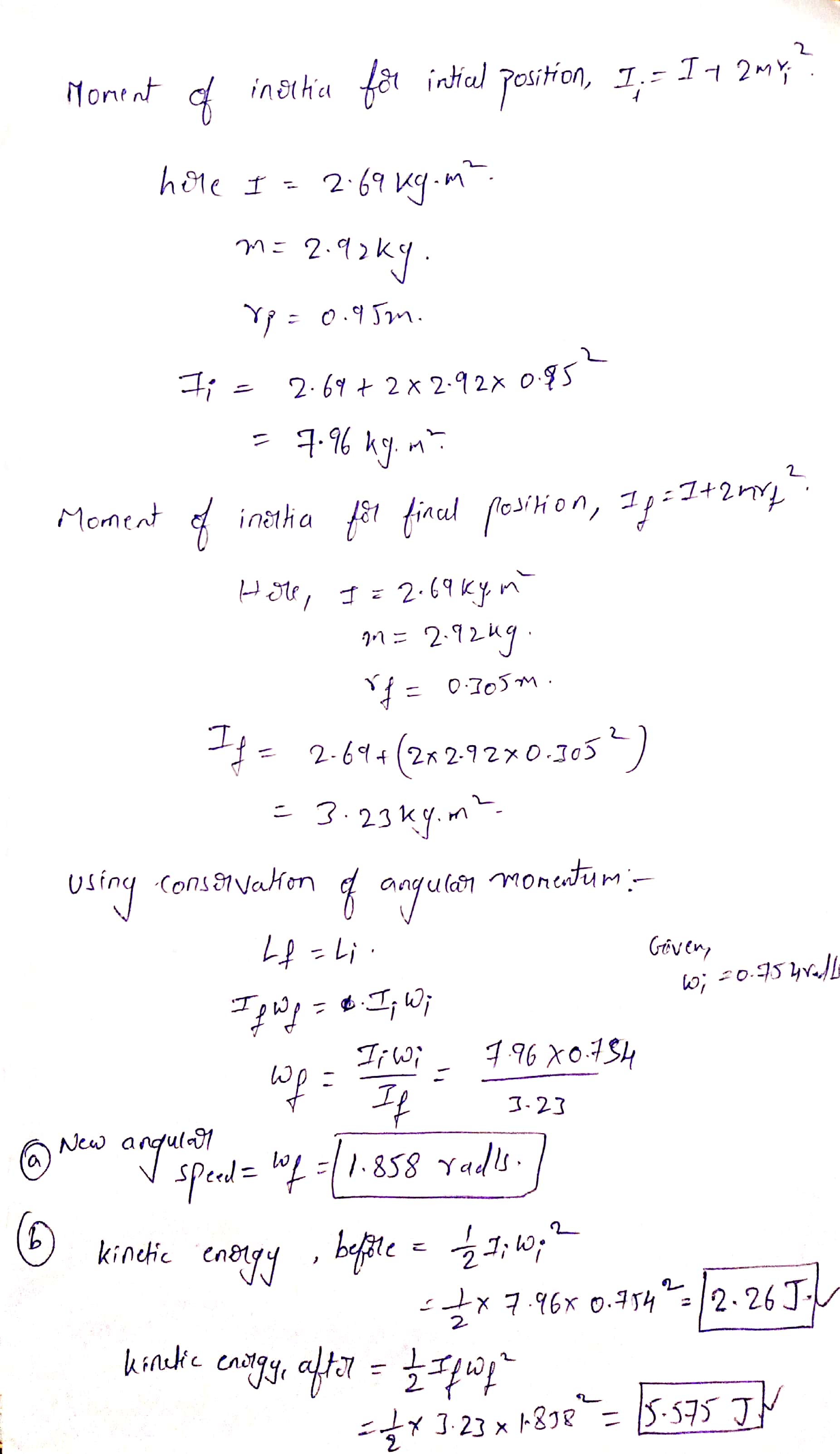#### Earn Coin

Coins can be redeemed for fabulous gifts.

Similar Homework Help Questions
• ### A student sits on a freely rotating stool holding two dumbbells, each of mass 2.98 kg...

A student sits on a freely rotating stool holding two dumbbells, each of mass 2.98 kg . When his arms are extended horizontally , the dumbbells are 0.96 m from the axis of rotation and the student rotates with an angular speed of 0.747 rad/s. The moment of inertia of the student plus stool is 2.62 kg · m2 and is assumed to be constant. The student pulls the dumbbells inward horizontally to a position 0.305 m from the rotation...

• ### A student sits on a freely rotating stool holding two dumbbells, each of mass 2.93 kg...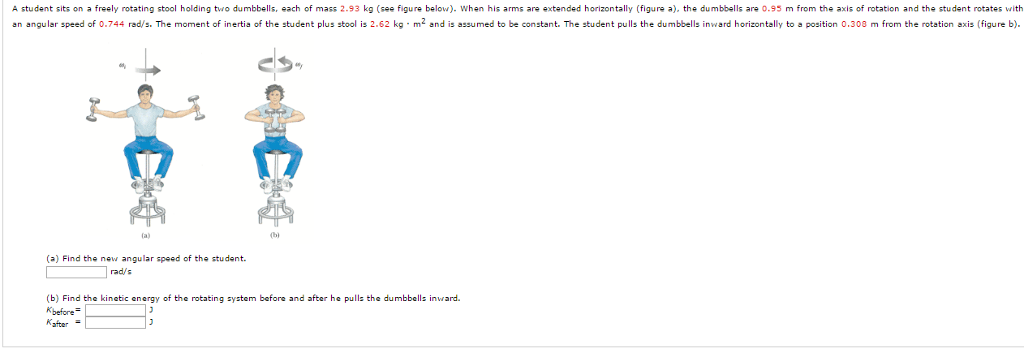A student sits on a freely rotating stool holding two dumbbells, each of mass 2.93 kg (see figure below). When his arms are extended horizontally (figure a), the dumbbells are 0.95 m from the axis of rotation and the student rotates with an angular speed of 0.744 rad/s. The moment of inertia of the student plus stool is 2.62 kg middot m^2 and is assumed to be constant. The student pulls the dumbbells inward horizontally to a position 0.308 m...

• ### A student sits on a freely rotating stool holding two dumbbells, each of mass 2.93 kg...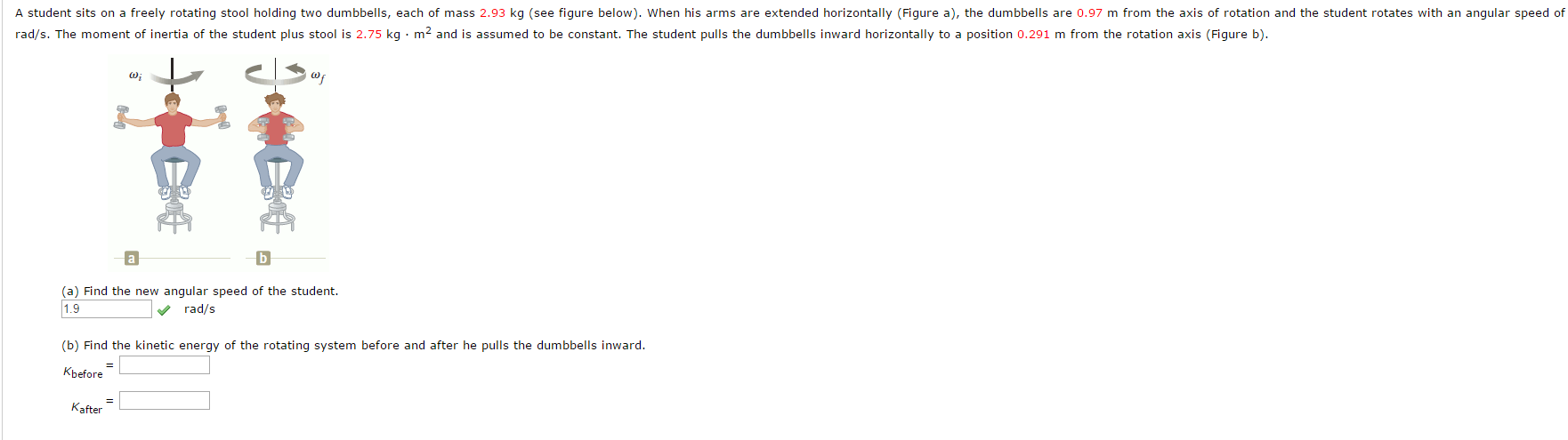A student sits on a freely rotating stool holding two dumbbells, each of mass 2.93 kg (see figure below). When his arms are extended horizontally (Figure a), the dumbbells are 0.97 m from the axis of rotation and the student rotates with an angular speed of rad/s. The moment of inertia of the student plus stool is 2.75 kg m^2 and is assumed to be constant. The student pulls the dumbbells inward horizontally to a position 0.291 m from the...

• ### A student sits on a freely rotating stool holding two dumbbells, each of mass 2.99 kg...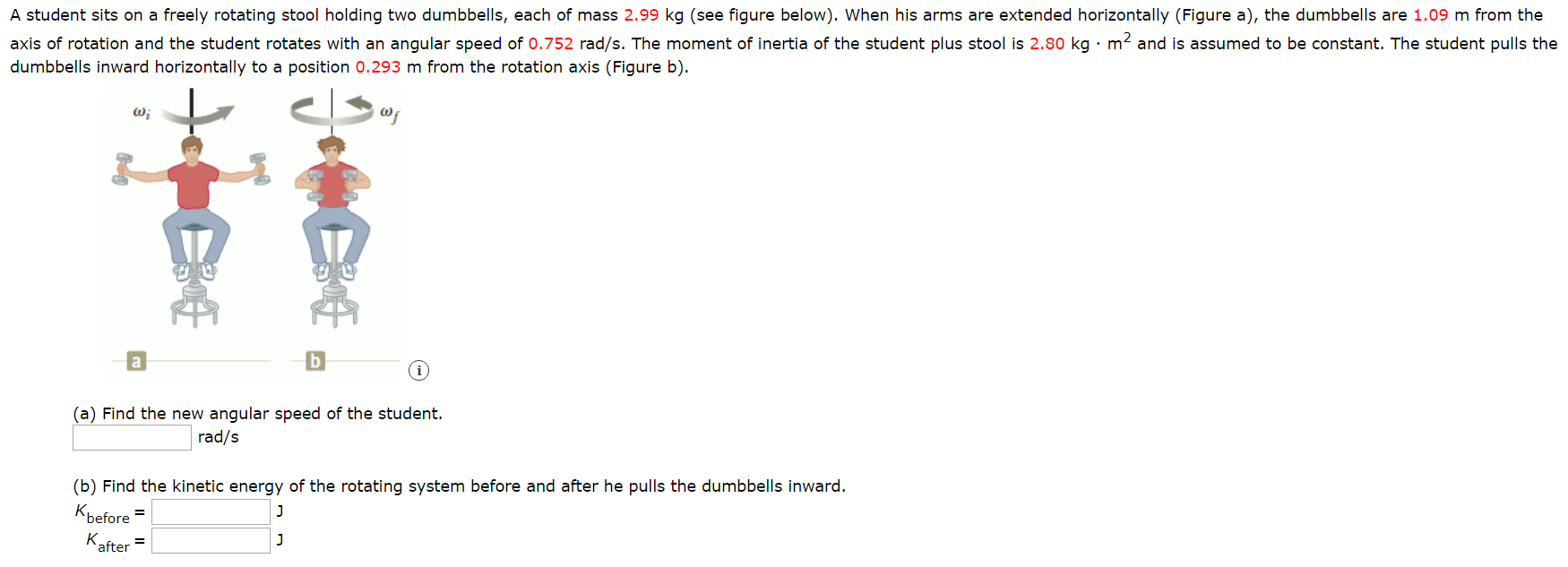A student sits on a freely rotating stool holding two dumbbells, each of mass 2.99 kg (see figure below). When his arms are extended horizontally (Figure a), the dumbbells are 1.09 m from the axis of rotation and the student rotates with an angular speed of 0.752 rad/s. The moment of inertia of the student plus stool is 2.80 kg . m and is assumed to be constant. The student pulls the dumbbells inward horizontally to a position 0.293 m...

• ### A student sits on a freely rotating stool holding two dumbbells, each of mass 3.08 kg...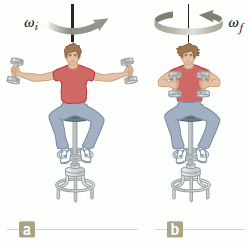A student sits on a freely rotating stool holding two dumbbells, each of mass 3.08 kg (see figure below). When his arms are extended horizontally (Figure a), the dumbbells are 0.96 m from the axis of rotation and the student rotates with an angular speed of 0.755 rad/s. The moment of inertia of the student plus stool is 2.53 kg · m2 and is assumed to be constant. The student pulls the dumbbells inward horizontally to a position 0.304 m...

• ### A student sits on a freely rotating stool holding two dumbbells, each of mass 3.04 kg...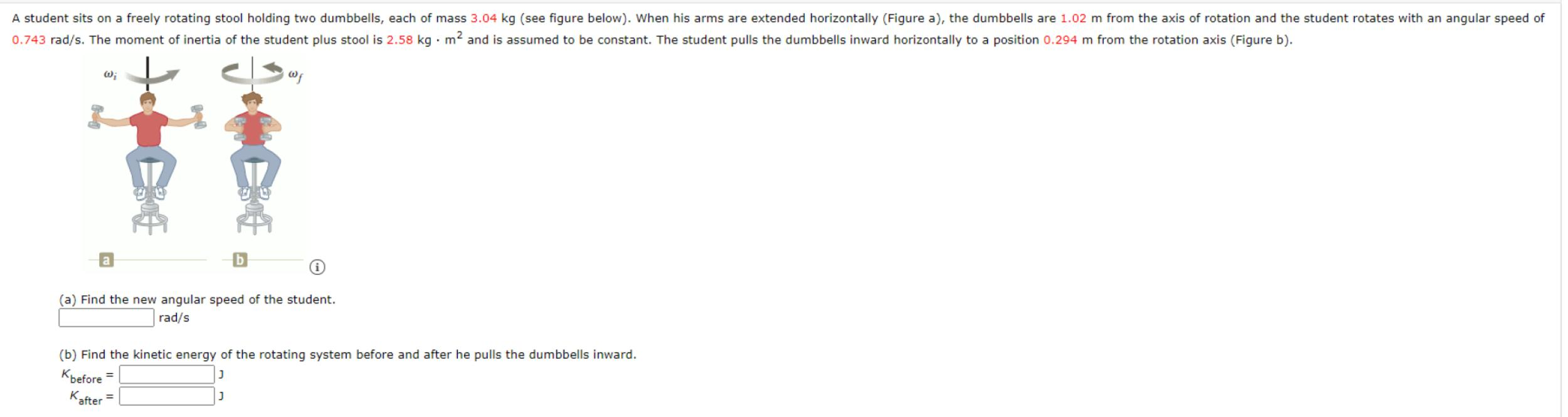A student sits on a freely rotating stool holding two dumbbells, each of mass 3.04 kg (see figure below). When his arms are extended horizontally (Figure a), the dumbbells are 1.02 m from the axis of rotation and the student rotates with an angular speed of 0.743 rad/s. The moment of inertia of the student plus stool is 2.58 kg .m2 and is assumed to be constant. The student pulls the dumbbells inward horizontally to a position 0.294 m from...

• ### A student sits on a freely rotating stool holding two dumbbells, each of mass 3.05 kg...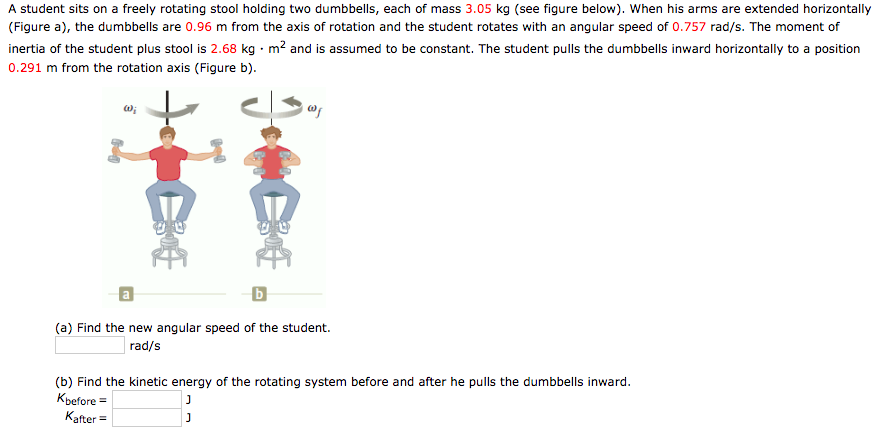A student sits on a freely rotating stool holding two dumbbells, each of mass 3.05 kg (see figure below). When his arms are extended horizontally (Figure a), the dumbbells are 0.96 m from the axis of rotation and the student rotates with an angular speed of 0.757 rad/s. The moment of inertia of the student plus stool is 2.68 kg m2 and is assumed to be constant. The student pulls the dumbbells inward horizontally to a position 0.291 m from...

• ### A student sits on a freely rotating stool holding two dumbbells, each of mass 3.04 kg...

A student sits on a freely rotating stool holding two dumbbells, each of mass 3.04 kg (see figure below). When his arms are extended horizontally (Figure a), the dumbbells are 1.08 m from the axis of rotation and the student rotates with an angular speed of 0.755 rad/s. The moment of inertia of the student plus stool is 2.59 kg · m2 and is assumed to be constant. The student pulls the dumbbells inward horizontally to a position 0.306 m...

• ### A student sits on a freely rotating stool holding two dumbbells, each of mass 2.96 kg...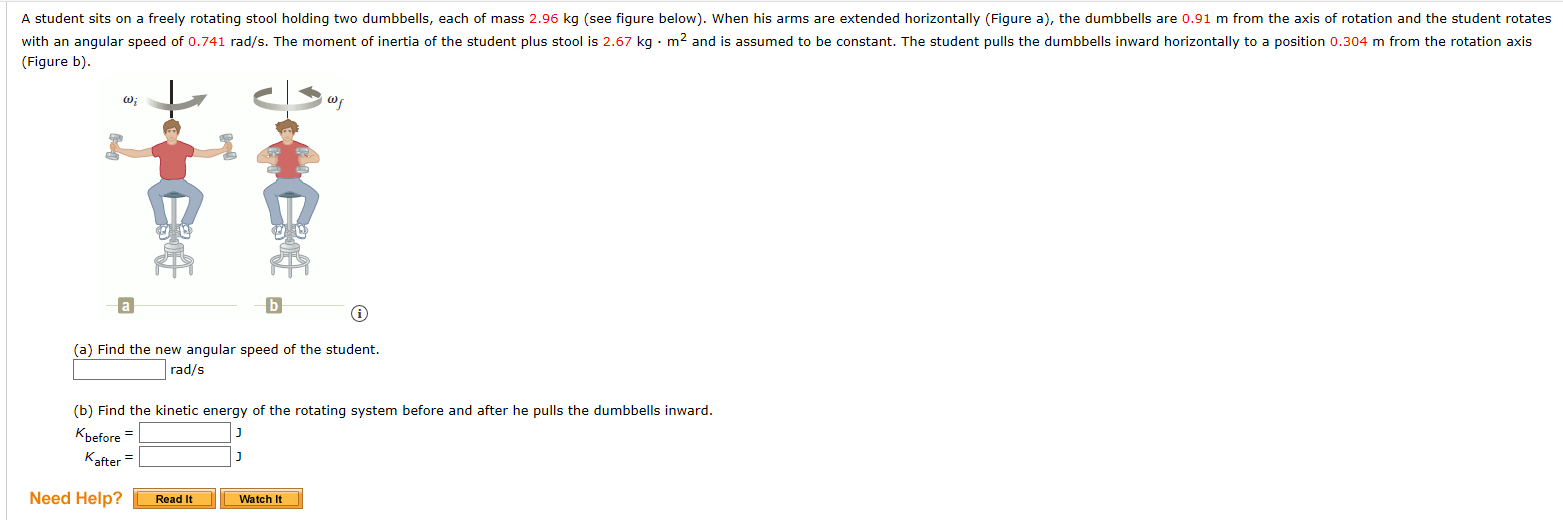A student sits on a freely rotating stool holding two dumbbells, each of mass 2.96 kg (see figure below). When his arms are extended horizontally (Figure a), the dumbbells are 0.91 m from the axis of rotation and the student rotates with an angular speed of 0.741 rad/s. The moment of inertia of the student plus stool is 2.67 kg. m2 and is assumed to be constant. The student pulls the dumbbells inward horizontally to a position 0.304 m from...

• ### A student sits on a freely rotating stool holding two weights, each of mass 4 kg.. When his arms are extended horizonta...

A student sits on a freely rotating stool holding two weights, each of mass 4 kg.. When his arms are extended horizontally, the weights are 1.1 m from the axis of rotation and he rotates with an angular speed of 0.9 rad/s. The moment of inertia of the student plus stool is 3.0 kg-m2 and is assumed to be constant. The student pulls the weights inward horizontally to a position 0.4 m from the rotation axis. Find the new angular...